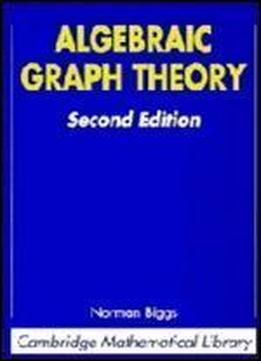# Algebraic Graph Theory (cambridge Mathematical Library) by Norman Biggs / 1974 / English / PDF

In this substantial revision of a much-quoted monograph first published in 1974, Dr. Biggs aims to express properties of graphs in algebraic terms, then to deduce theorems about them. In the first section, he tackles the applications of linear algebra and matrix theory to the study of graphs algebraic constructions such as adjacency matrix and the incidence matrix and their applications are discussed in depth. There follows an extensive account of the theory of chromatic polynomials, a subject that has strong links with the "interaction models" studied in theoretical physics, and the theory of knots. The last part deals with symmetry and regularity properties. Here there are important connections with other branches of algebraic combinatorics and group theory. The structure of the volume is unchanged, but the text has been clarified and the notation brought into line with current practice. A large number of "Additional Results" are included at the end of each chapter, thereby covering most of the major advances in the past twenty years. This new and enlarged edition will be essential reading for a wide range of mathematicians, computer scientists and theoretical physicists

views: 696